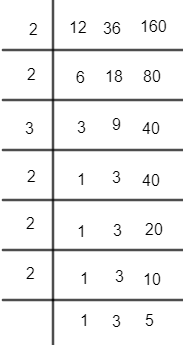QuestionAnswers

# Find the LCM of 12, 36 and 160 by prime factorization method.Hint: By using prime factorization, split the given number as a product of prime numbers. Thus, from three prime numbers find the LCM taking the common term together and multiply together the rest of the terms.

The method of prime factorization is used to break down or express a given number as a product of prime numbers. More so, if a prime number occurs more than once in the factorization, it is usually expressed in exponential form to make it look more compact. Here, a prime number is a whole number greater than 1 that is only divisible by 1 and itself. Another way to say it is that a prime number has exactly two factors, namely 1 and itself. Let us explain prime factorization in steps.
First let us list down a few prime numbers: 2, 3, 5, 7, 11, 13, 17, etcetera.
Now, we have been asked to find the LCM of 12, 36 and 160.
Let us check again if the numbers are divisible by the prime number 2. After the repeated division of 2, you end up getting a prime number. Thus, we can form the prime factorization as,
$12=2\times 2\times 3$
$36=2\times 2\times 3\times 3$
$160=2\times 2\times 2\times 2\times 2\times 5$
Thus, to find the LCM from the above $\left( 2\times 2 \right)=4$ is common for the three numbers 12, 36 and 160.
Thus, we can write the LCM as (4 $\times$ the rest of the prime numbers)
$\therefore LCM=4\times 3\times 3\times 3\times 2\times 2\times 2\times 5=4320$
Thus, we got the LCM $=4320$ .

Note: We can also find the LCM using the division method.$\therefore LCM=2\times 2\times 3\times 3\times 2\times 2\times 2\times 3\times 5$
$={{2}^{5}}\times {{3}^{2}}\times 5=4320$
View Notes
Prime Factorization Of HCF And LCMApplication of LCM and HCFSquare Root Prime FactorizationLCM FormulaFactors of 36Table of 36 - Multiplication Table of 36Cube Root by Factorization MethodCBSE Class 12 Maths Chapter-8 Application of Integrals FormulaPrime NumbersCBSE Class 8 Maths Chapter 12 - Exponents and Powers FormulasCBSE Class 8 Science Conservation of Plants and Animals WorksheetsCBSE Class 8 Science Reaching The Age of Adolescence WorksheetsCBSE Class 8 Science Pollution of Air and Water WorksheetsCBSE Class 8 Science Stars and The Solar System WorksheetsImportant Questions for CBSE Class 12 Maths Chapter 8 - Application of IntegralsImportant Questions for CBSE Class 8 Social Science - Social and Political Life Chapter 8 - Confronting MarginalisationImportant Questions for CBSE Class 8 Maths Chapter 12 - Exponents and PowersImportant Questions for CBSE Class 12 Chemistry Chapter 8 - The d and f Block ElementsCBSE Class 8 Science Force and Pressure WorksheetsCBSE Class 8 Science Combustion and Flame WorksheetsCBSE Class 12 Maths Question Paper 2020Maths Question Paper for CBSE Class 12 - 2013Previous Year Question Paper of CBSE Class 10 EnglishPrevious Year Question Paper for CBSE Class 12 Maths - 2014CBSE Previous Year Question Papers Class 12 Maths with SolutionsMaths Question Paper for CBSE Class 12 - 2016 Set 1 CMaths Question Paper for CBSE Class 12 - 2016 Set 1 EMaths Question Paper for CBSE Class 12 - 2016 Set 1 SCBSE Class 12 Maths Question Paper 2018 with Solutions - Free PDFCBSE Class 12 Maths Question Paper 2015 with Solutions - Free PDFNCERT Solutions for Class 8 Maths Chapter 12 Exponents and PowersNCERT Solutions for Class 6 Maths Chapter 8NCERT Solutions for Class 12 ChemistryCell Structure and Functions NCERT Solutions - Class 8 ScienceNCERT Solutions for Class 12 Biology Chapter 8RD Sharma Class 12 Solutions Chapter 8 - Solution of Simultaneous Linear Equations (Ex 8.2) Exercise 8.2RD Sharma Class 10 Solutions Chapter 8 - Exercise 8.12RD Sharma Class 12 Solutions Chapter 8 - Solution of Simultaneous Linear Equations (Ex 8.1) Exercise 8.1NCERT Solutions for Class 8 Social Science - Social and Political Life Chapter-8NCERT Solutions for Class 8 Maths Chapter 7 Cubes and Cube Roots